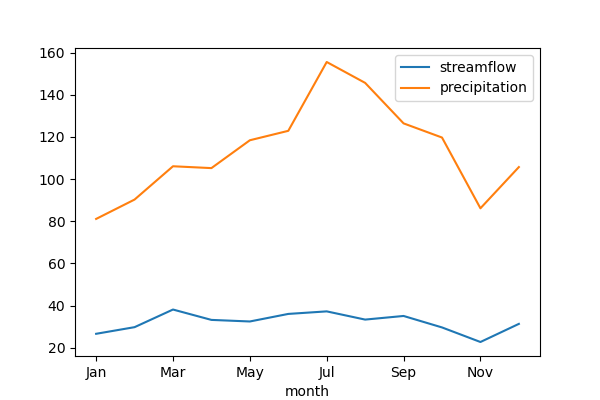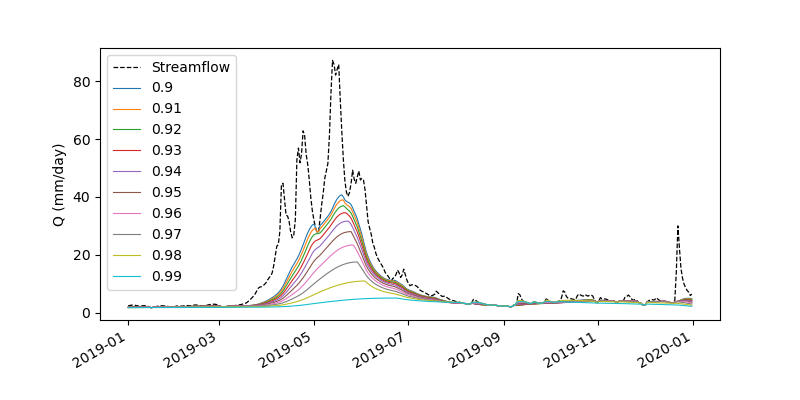# HydroSignatures: Tools for computing hydrological signatures## Features#

HydroSignatures is a suite of tools for computing hydrological signatures and a part of HyRiver software stack. This package includes the following functions:

• `compute_exceedance`: Exceedance probability that can be used plotting flow duration curves;

• `compute_mean_monthly`: Mean monthly summary of a time series that can be used for plotting regime curves;

• `compute_rolling_mean_monthly`: Rolling mean monthly summary of a time series that can be used for plotting smoothed regime curves;

• `compute_baseflow`: Extracting baseflow from a streamflow time series using the Lyne and Hollick digital filter (Ladson et al., 2013);

• `compute_bfi`: Baseflow index;

• `compute_ai`: Aridity index;

• `compute_si_walsh`: Seasonality index (Walsh and Lawler, 1981);

• `compute_si_markham`: Seasonality index (Markham, 1970);

• `extract_extrema`: Determining the location of local maxima and minima in a time series;

Moreover, the package has a class called `HydroSignatures` that can be used to compute all these signatures by passing a streamflow and a precipitation time series, both in millimeters per day (or any other unit of time). This class supports subtraction and inequality operators, which can be used to compare two `HydroSignatures` objects. You can serialize the class to a JSON object using the `to_json` method or convert it to a dictionary using the `to_dict` method.

Moreover, `numba` is an optional dependency for the `compute_baseflow` function. Installing `numba` will speed up the computation of baseflow significantly.

You can also try using HydroSignatures without installing it on your system by clicking on the binder badge. A Jupyter Lab instance with the HyRiver stack pre-installed will be launched in your web browser, and you can start coding!

Moreover, requests for additional functionalities can be submitted via issue tracker.

# Citation#

If you use any of HyRiver packages in your research, we appreciate citations:

```@article{Chegini_2021,
author = {Chegini, Taher and Li, Hong-Yi and Leung, L. Ruby},
doi = {10.21105/joss.03175},
journal = {Journal of Open Source Software},
month = {10},
number = {66},
pages = {1--3},
title = {{HyRiver: Hydroclimate Data Retriever}},
volume = {6},
year = {2021}
}
```

# Installation#

You can install HydroSignatures using `pip`:

```\$ pip install hydrosignatures
```

or from the `conda-forge` repository using Conda or Mamba:

```\$ conda install -c conda-forge hydrosignatures
```

# Quick start#

Let’s explore the capabilities of `HydroSignatures` by getting streamflow using PyGeoHydro, basin geometry using PyNHD and precipitation using PyDaymet. In this example, we select West Branch Herring Run At Idlewylde, MD, as the watershed of interest and compute the hydrological signatures for the period from 2010 to 2020.

```import pydaymet as daymet
import hydrosignatures as hs
import pygeohydro as gh
from hydrosignatures import HydroSignatures
from pygeohydro import NWIS
from pynhd import WaterData

site = "01585200"
start = "2010-01-01"
end = "2020-12-31"
```

First, we get the basin geometry of the watershed using `gagesii_basins` layer of the USGS’s WaterData web service.

```wd = WaterData("gagesii_basins")
geometry = wd.byid("gage_id", site).geometry
```

Then, we obtain the station’s info and streamflow data using NWIS. Note that we should convert the streamflow from cms to mm/day.

```nwis = NWIS()
info = nwis.get_info({"site": site})
area_sqm = info.drain_sqkm.values * 1e6
q_cms = nwis.get_streamflow(site, (start, end))
q_mmpd = q_cms * (24.0 * 60.0 * 60.0) / area_sqm * 1e3
q_mmpd.index = pd.to_datetime(q_mmpd.index.date)
```

Next, we retrieve the precipitation data using PyDaymet over the whole basin using the basin geometry and take its mean as the basin’s precipitation.

```prcp = daymet.get_bygeom(geometry, (start, end), variables="prcp")
p_mmpd = prcp.prcp.mean(dim=["x", "y"]).to_pandas()
p_mmpd.index = pd.to_datetime(p_mmpd.index.date)
q_mmpd = q_mmpd.loc[p_mmpd.index]
```

Now, we can pass these two to the `HydroSignatures` class:

```sig = HydroSignatures(q_mmpd, p_mmpd)
```

The `values` property of this class contains the computed signatures. For example, let’s plot the regime curves:

```sig.values.mean_monthly.plot()
```Note that, you can also use the functions directly. For example, let’s get streamflow observations for another station and separate the baseflow using various filter parameters and compare them:

```import numpy as np
import pandas as pd

q = nwis.get_streamflow("12304500", ("2019-01-01", "2019-12-31"))
alpha = np.arange(0.9, 1, 0.01)
qb = pd.DataFrame({a: hs.compute_baseflow(q.squeeze(), alpha=a) for a in alpha})
```Lastly, let’s compute Markham’s seasonality index for all streamflow time series of the stations in the CAMELS dataset. We retrieve the CAMELS dataset using PyGeoHydro:

```import xarray as xr

_, camels_qobs = gh.get_camels()
discharge = camels_qobs.discharge.dropna("station_id")
discharge = xr.where(discharge < 0, 0, discharge)
si = hs.compute_si_markham(discharge.to_pandas())
```

More examples can be found here.